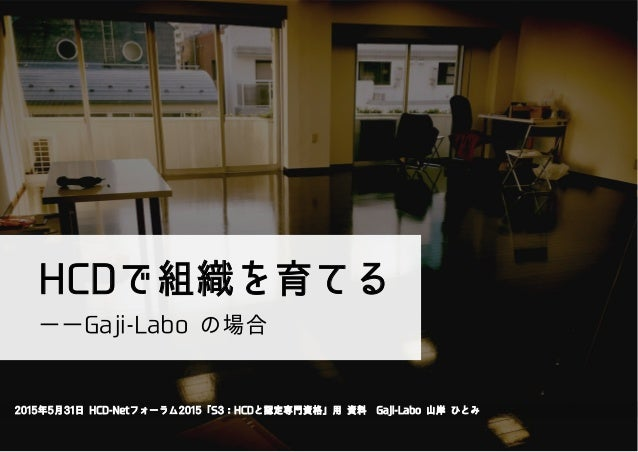Successfully reported this slideshow.Upcoming SlideShare
×

# HCDで組織を育てる ーーGaji-Laboの場合

1,458 views

Published on

2015年5月31日、HCD-Netフォーラム2015「S3：HCDと認定専門資格」での発表用資料。

Published in: Design
• Full Name
Comment goes here.

Are you sure you want to Yes No• Be the first to comment

### HCDで組織を育てる ーーGaji-Laboの場合

1. 1. HCDで組織を育てる  2015年5月31日 HCD-Netフォーラム2015「S3：HCDと認定専門資格」用 資料 Gaji-Labo 山岸 ひとみ ――Gaji-Labo の場合
2. 2. W 16 : 367 /54
3. 3. システム プロセス ユーザー 設計／デザイン C h C w p D g x g D 9 . 86 : -‐‑‒! r j C
4. 4. •  C × •  × GL G G L •  •  wL •  wL •  -‐‑‒! r j C
5. 5. -‐‑‒! ×   × × × × W G W G O O j o j o
6. 6. -‐‑‒! W a L h i i r j C
7. 7. -‐‑‒! W a L
8. 8. L •  g •  bg h g •  i •  t •  •  h g •  X h g
9. 9. L •  t j •  bg •  X h •  r L •  Xw j
10. 10. -‐‑‒!
11. 11. •  s p i W •  Hj h h g •  hg g •  Hj t h g •  j C g
12. 12. •  w g •  j j •  •
13. 13. -‐‑‒! W a L r j C C j Hj C g w
14. 14. b GLk W G D
15. 15. ×   × × × × W G W G O O j o -‐‑‒! j o pr W C pr C W
16. 16. 1
17. 17. pr b x h
18. 18. pr
19. 19. pr 0: :
20. 20. pr w
21. 21. pr jg
22. 22. pr 2/0o
23. 23. h h H
24. 24. ×   × × × × W G W G O O j o -‐‑‒! j o W W a
25. 25. W pr g
26. 26. W pr X
27. 27. W pr
28. 28. W pr
29. 29. -‐‑‒! D -‐‑‒ × × × × -‐‑‒× W D j GD jL D
30. 30. papa j X i
31. 31. b a W r W C G W
32. 32. ISO9241-210 HCD x
33. 33. C j a a G
34. 34. x h p G